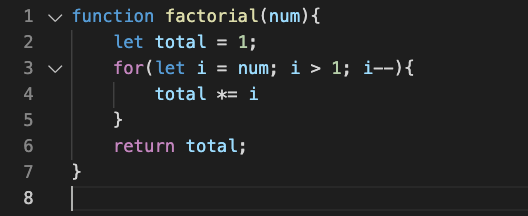# Recursion

• A base case, or condition that is checked previous to execution (if this condition isn’t present the function call loop would run forever, but would lead to a call stack overflow error).
• A different input on each function call. This is what makes recursive functions make sense. If we always provided the same input to the function call, we wouldn’t obtain a different output.We have a helper variable that starts at 1, and a loop that multiplies the input number with the helper variable and subtracts 1 to the input number.

--

--